# Dice

The concepts and questions on Dice might mystify some people but it can be a cakewalk for the people with a great visual bent of mind and visualization. It’s an extension of Cubes but with a slightly higher logic and conceptual application.

Dice is a part of the logical reasoning section of aptitude. The frequency of the appearance of questions on dice is high every year.

Following is the list of exams where the questions on dice are frequent and evident in all the question papers.

 List of Competitive Govt Exams SBI PO IBPS PO SBI Clerk IBPS SO SBI SO IBPS Clerk Staff Selection Commission (SSC) IBPS Regional Rural Banks (RRB)

## What is a dice?

Dice is a cube having different numbers on all its faces. The numbers are usually a single digit, from 1 to 6.

To explore various SBI Exams at length, check at the linked article.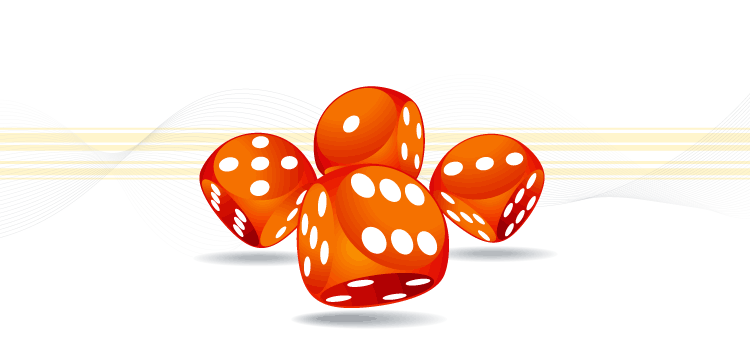A schematic representation of dice with numbers

### Numbers on a die:

The scheme of numbering on a die is specific. The sum of the number on the opposite faces will be equal to ‘7’ always. The number 1 will be opposite to number 6. The number 2 is opposite to 5 and 3 is opposite to 4. Such that:

1. 1 + 6 = 7
2. 2 + 5 = 7
3. 3 + 4 =7.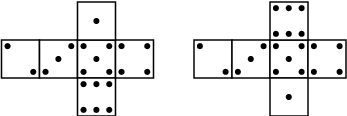Numbering scheme of a dice

### Classification of dice:

There are two types of dice:

1. Base dice: The base dice is further classified into two types.
2. Standard dice
3. Ordinary dice

When the dice are rolled, if the number on the faces of the two dice do not match each other, they are called as standard dice.

Example for standard dice: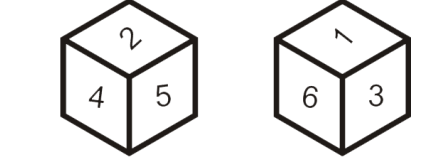If one or more than one number matches between two dice than it is called as an ordinary dice.

Example: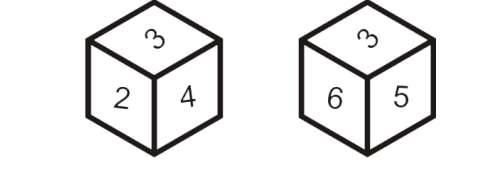In the above dice, the number ‘3’ is common in both the dice.

1. Open dice: In an open dice all the six faces of the dice are shown. The dices show the opposite position of rows and columns.

Example: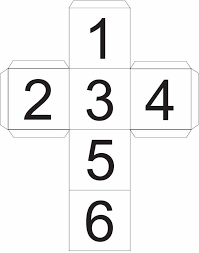A schematic representation of an open die.

To explore Logical Reasoning in detail, check at the linked article.

### Constructed and Deconstructed Dice:

Constructed dice: This section of dice gives the constructed version of dice, the questions will be based on the visualization of the flattened-out version of dice.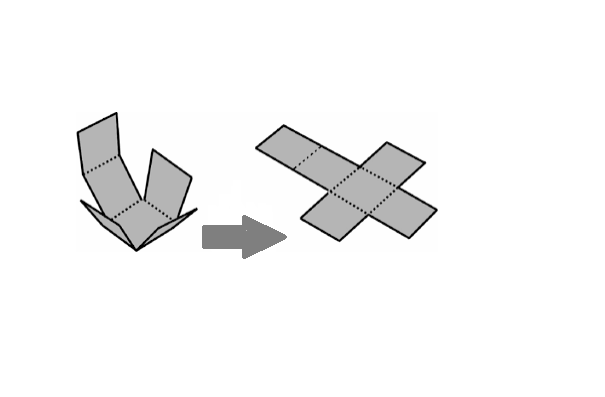Conversion of constructed to flat end die

Deconstructed Dice: This section of dice gives the flattened-out version of dice, the questions will be based on the visualization of what is at the other end of the constructed dice.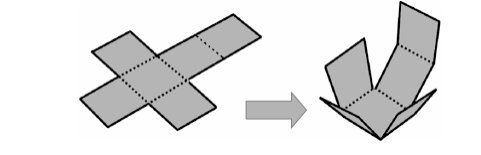Conversion of flat end to constructed die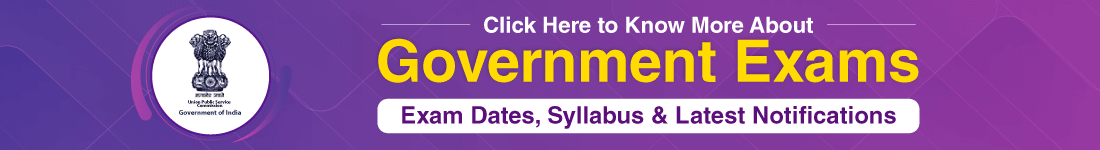Rules to remember

There are a few rules that will help in solving the questions on dices in the easiest way. The rules are as follows:

Rule #1:

If one of the numbers is common in both the dice when two dice have the same surface, then the remaining surfaces of both dice are opposite to each other.

Example: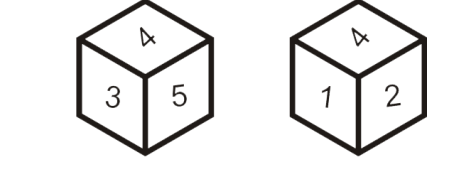In the above two dice, the number 4 is common and the numbers 3 and 5 on one die and 1 and 2 on the surface of the other die are opposite to each other respectively. I.e. The number 3 is opposite 1 and 5 is opposite to 2.

Rule #2:

If any two numbers are the same in two dices irrespective of their position on the dice then the remaining third number in both the dice are opposite to each other.

Example: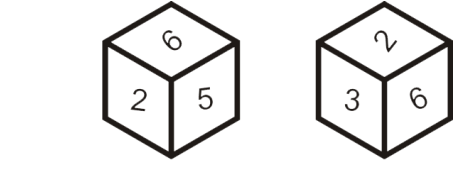In the above scenario, irrespective of their place or position the two numbers 6 and 2 are common in both the dice. Then the third number 5 on one first die and 3 on the second die are opposite to each other.

Rule #3:

If there is one element common on both the dice at the different position, then rotate the dice in a clockwise direction to get the opposite surface.

Example: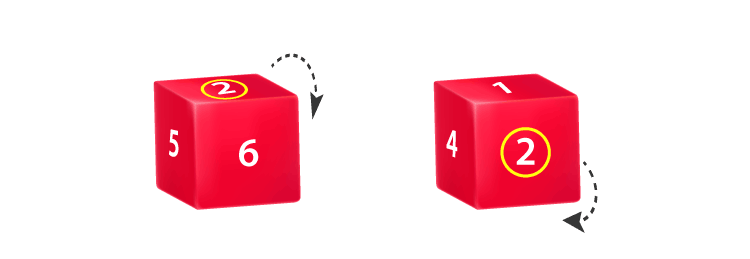The number ‘2’ is common in both the dice, but it is occurring at a different position. In order to find out, the number appearing on the opposite face, rotate the die in a clockwise direction with the common number as the reference.

To explore Directions in detail, check at the linked article.

The rotation of the first die in the clockwise direction gives ‘6’ at the front face. Now the rotation of the second die in the clockwise direction gives ‘4’ in the front face. Hence the number ‘6’ is opposite to ‘4’. A similar operation gives the result that the number ‘5’ is opposite to ‘1’.This also indicates that the number ‘3’ is opposite to ‘2’.

An Open die: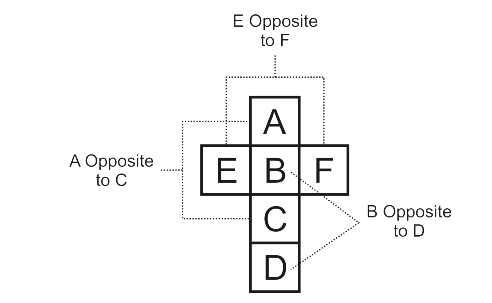A flat end version of a die

Observations of an open die:

The faces E & F, A & C, B & D are opposite to each other and their surfaces never touch each other.

Variations of open dice

 Open die Opposite faces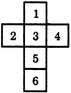1 is opposite to 5. 2 is opposite to 4. 3 is opposite to 6.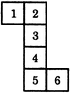1 is opposite to 6 2 is opposite to 4 3 is opposite to 5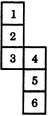1 is opposite to 3 2 is opposite to 5 4 is opposite to 6

Example 1: Possible combinations of die.

Observe the open die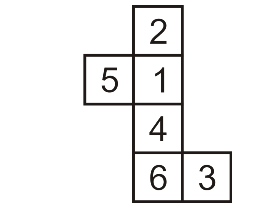The number of a possible combination is: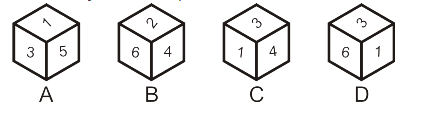1. Only A
2. Only B
3. Only C
4. Only D

Solution:

Option 1 is not possible as 3 is not opposed to 5. The second option is also wrong as 2 is not adjacent to 4. Option 3 is possible. And option 4 is not possible as 1 is not adjacent to 6.

Hence the correct answer is option 3 i.e. only C.

To explore Calendars in detail, check at the linked article.

Example 2: Finding the opposite side.

Observe the die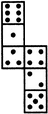If the surfaces of the above die are reconstructed to form a perfect die. How many dots lie opposite to the face having three dots?

A.2

B.4

C.5

D.6

Solution:

Since 4, 5 and 2 are adjacent to 3, six is the only possibility.

Hence the correct answer is option D.

To explore Seating Arrangement in detail, check at the linked article.

Example 3:

Choose the die that is similar to the die, formed from the open die.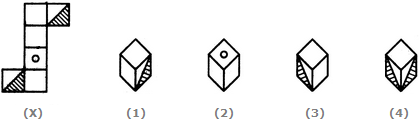1. Only 1
2. Only 2
3. 1 and 3
4. 1, 2 and 3

Solution:

The figure number 1 is possible as the top face is shaded and adjacent faces are blank. Figure 2 is not possible as the surface with a dot should lie between shaded one, but here it is blank. Figure 3 is possible but the 4th one is impossible as the two shaded faces cannot be adjacent to each other. Hence, the correct answer is option C.

Important Bank/Govt Information:Example 4:

Observe the dice

How many dots are contained on the face opposite to that containing four dots?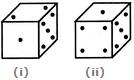1. 1
2. 2
3. 3
4. 6

Solution:

From the second figure, it can be observed that the face with 4 dots is adjacent to the one with 1 and 5 dots. Hence, then comparing this with the first die it can see that the only place possible the face with 4 dots is opposite it to the face having 2 dots. Hence the correct answer is option B.

Example 5:

Observe the dice.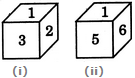Two positions of a dice are shown. When 4 is at the bottom, what number will be on the top?

A.1

B.2

C.5

D.6

Solution:

It can be observed from both the dice, if 2,3,5 and 6 adjacent to 1. Then the number opposite 1 should be 4. Hence the correct answer is option A.

To explore Clocks in detail, check at the linked article.

Example 6:

A dice is numbered from 1 to 6 in different ways. If 1 is adjacent to 2, 3 and 5, then which of the following statements is necessarily true?